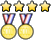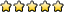Hovercraft B/C

Test your knowledge of various Science Olympiad events.
photolithoautotroph
MemberPosts: 15
Joined: April 8th, 2018, 6:21 pm
Division: C
State: PA

Re: Hovercraft B/C

Consider a pipe with radius r.

If an ideal fluid is flowing through the pipe, how would the flow rate change if the radius was increased to 2r?

If a non-ideal, viscous fluid is flowing through the pipe, how would the flow rate change if the radius was increased to 2r?
Both fluids would go through twice as slowly?
I haven't done enough fluid dynamics to be sure, but I can guess: for an ideal fluid the flow rate would change to 4Q (Q=Av) and for a non-ideal, viscous fluid, the flow rate would change to 16Q (Poiseuille's Law)? Less sure about the second one but either could be wrong. At least I don't actually have to do this for real.
i wish i was goodEvents 2019: Expd, Water, Herp
Rip states 2019

Tesel
Exalted MemberPosts: 161
Joined: January 30th, 2016, 8:03 pm
Division: C
State: MI

Re: Hovercraft B/C

Consider a pipe with radius r.

If an ideal fluid is flowing through the pipe, how would the flow rate change if the radius was increased to 2r?

If a non-ideal, viscous fluid is flowing through the pipe, how would the flow rate change if the radius was increased to 2r?
Both fluids would go through twice as slowly?
I haven't done enough fluid dynamics to be sure, but I can guess: for an ideal fluid the flow rate would change to 4Q (Q=Av) and for a non-ideal, viscous fluid, the flow rate would change to 16Q (Poiseuille's Law)? Less sure about the second one but either could be wrong. At least I don't actually have to do this for real.
I should have clarified the question a lot better.
But yes, you made the right assumptions, and that was the correct use of Poiseuille's Law. Correct!
University of Michigan Science Olympiad Div. C Event Lead

2018 MI Mission Possible State Champions

CMS AC
MemberPosts: 151
Joined: January 10th, 2017, 3:54 pm
Division: B
State: NJ

Re: Hovercraft B/C

Consider a pipe with radius r.

If an ideal fluid is flowing through the pipe, how would the flow rate change if the radius was increased to 2r?

If a non-ideal, viscous fluid is flowing through the pipe, how would the flow rate change if the radius was increased to 2r?
Both fluids would go through twice as slowly?
I haven't done enough fluid dynamics to be sure, but I can guess: for an ideal fluid the flow rate would change to 4Q (Q=Av) and for a non-ideal, viscous fluid, the flow rate would change to 16Q (Poiseuille's Law)? Less sure about the second one but either could be wrong. At least I don't actually have to do this for real.

photolithoautotroph
MemberPosts: 15
Joined: April 8th, 2018, 6:21 pm
Division: C
State: PA

Re: Hovercraft B/C

If nobody posts a question in this long, you can.
Derive a formula for the acceleration of the blocks in a real atwood machine with two masses of mass $m1$ and $m2$and a pulley in the shape of a uniform disk of mass $M$ and radius $r$. The string is massless. Ignore friction.
i wish i was goodEvents 2019: Expd, Water, Herp
Rip states 2019

IvanGe
MemberPosts: 196
Joined: September 29th, 2016, 1:56 pm
Division: B
State: NY
Location: Ward Melville

Re: Hovercraft B/C

If nobody posts a question in this long, you can.
Derive a formula for the acceleration of the blocks in a real atwood machine with two masses of mass $m1$ and $m2$and a pulley in the shape of a uniform disk of mass $M$ and radius $r$. The string is massless. Ignore friction.
a = m1g - m2g / m1+ m2
gelinas 2016-2019, wmhs'22

nats '19:
5th - potions
5th - fossils
9th - buggy

UTF-8 U+6211 U+662F
Exalted MemberPosts: 1518
Joined: January 18th, 2015, 7:42 am
Division: C
State: PA

Re: Hovercraft B/C

If nobody posts a question in this long, you can.
Derive a formula for the acceleration of the blocks in a real atwood machine with two masses of mass $m1$ and $m2$and a pulley in the shape of a uniform disk of mass $M$ and radius $r$. The string is massless. Ignore friction.
The acceleration of m1 where positive is down is
EDIT: Whoops, didn't see other answer

PW240.3
MemberPosts: 1
Joined: May 7th, 2018, 2:14 pm
Division: B
State: TX

Re: Hovercraft B/C

If nobody posts a question in this long, you can.
Derive a formula for the acceleration of the blocks in a real atwood machine with two masses of mass $m1$ and $m2$and a pulley in the shape of a uniform disk of mass $M$ and radius $r$. The string is massless. Ignore friction.

Since you have to account for the pulley's moment of inertia, wouldn't the a be a=(m1-m2)g/(m1+m2+M/4)

IvanGe
MemberPosts: 196
Joined: September 29th, 2016, 1:56 pm
Division: B
State: NY
Location: Ward Melville

Re: Hovercraft B/C

I'm just going to ask a question because no one's verified the answer.

With what constant velocity can a 4.00 hp motor raise a mass of 125 kg?
gelinas 2016-2019, wmhs'22

nats '19:
5th - potions
5th - fossils
9th - buggy

ModeratorPosts: 473
Joined: December 6th, 2013, 1:56 pm
State: TX
Location: Austin, Texas

Re: Hovercraft B/C

I'm just going to ask a question because no one's verified the answer.

With what constant velocity can a 4.00 hp motor raise a mass of 125 kg?
2.43 meters per second
University of Texas at Austin '22
Seven Lakes High School '18
Beckendorff Junior High '14

IvanGe
MemberPosts: 196
Joined: September 29th, 2016, 1:56 pm
Division: B
State: NY
Location: Ward Melville

Re: Hovercraft B/C

I'm just going to ask a question because no one's verified the answer.

With what constant velocity can a 4.00 hp motor raise a mass of 125 kg?
2.43 meters per second

that's what i got, but the answer key said 2.76 m/s?